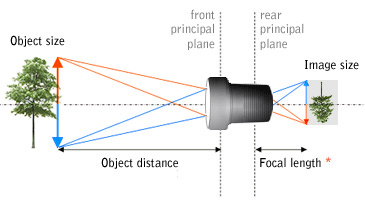Please note: All calculations are based on theoretical formulas only, assuming the principal planes are identical. Actual results will differ! Our sales team will be happy to assist you!

# Focal Length Calculator

With this tool you can calculate the required focal length to take a picture of an object at a given distance in order to generate an image fitting on your sensor size.

 Object size: mm Image size: 1/4" 3.61/3" 4.81/2.5" 5.81/2" 6.42/3" 8.81" 12.8 mm Object distance: m Magnification: x Focal length: mm Angle of view: °Of course, all distances are measured from the lens front and rear principal points respectively. Too often, unfortunately, these points are not specified by the lens manufacturer: it's hard to guess their position, since they can be anywhere inside or outside the lens. Anyway, the rear principal point is always one focal length ahead the sensor * when the lens is focused at infinity. The location of the front principal point cannot be neglected when using macro lenses.

# Depth of field calculators

This page is useful to calculate the depth of field when using a given lens or to choose the required aperture in order to achieve a given depth of field.

The circle of confusion c is the diameter of a circle on the image plane that can be considered a single point when the image is observed (the size of the film/imager is different that the size of the print/screen. For 35 mm photography c is usually 0.025 mm, but this value depends of course on the size of the final prints.

The hyperfocal distance H is the minimum distance from the front principal plane that will appear sharp when the lens is focuses at infinity (xo = infinity).

Calculator for finding the depth of field
for a given lens and aperture.

 Focal length: f = mm Aperture: N = f / Object distance: xo = m Circle of confusion: c = mm Hyperfocal distance: H = m Far depth of field: Df = m Near depth of field: Dn = m Depth of field: D = m

Calculator for finding the required aperture
for a given depth of field and a given lens.

 Focal length: f = mm Far depth of field: Df = m Near depth of field: Dn = m Circle of confusion: c = mm Aperture: N = f / Object (focus) distance: xo = m Hyperfocal distance: H = m

The maximum possible depth of field is obtained when the lens is focused one focal length f farther than the hyperfocal distance H: everything between Dn and infinity will be sharp. The value "Infinity" can be entered for Df, but since it's case sensitive it has to be typed as shown.